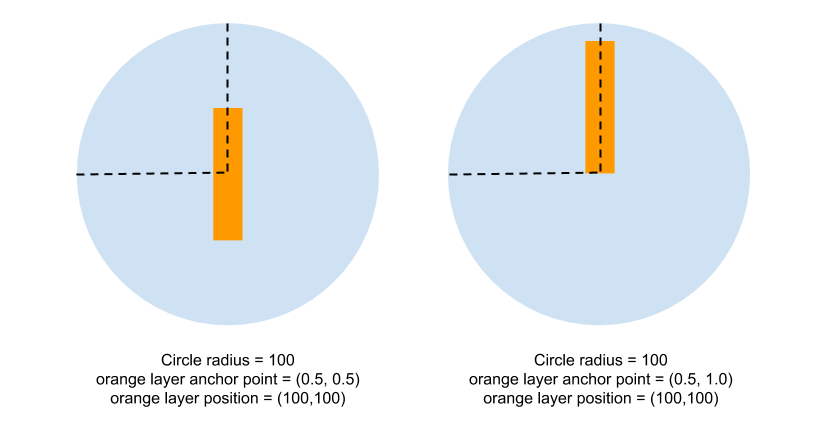# Understanding CALayer anchorPoint and position property

For me understanding `position` and `anchorPoint` was easiest when I started comparing it with my understanding of frame.origin in UIView. A UIView with frame.origin = (20,30) means that the UIView is 20 points from left and 30 points from top of its parent view. This distance is calculated from which point of a UIView? Its calculated from top-left corner of a UIView.

In layer `anchorPoint` marks the point (in normalized form or unit-coordinates i.e. 0 to 1) from where this distance is calculated so e.g. layer.position = (20, 30) means that the layer `anchorPoint` is 20 points from left and 30 points from top of its parent layer. By default a layer anchorPoint is (0.5, 0.5) so the distance calculation point is right in the center of the layer. The following figure will help clarify my point:`anchorPoint` also happens to be the point around which rotation will happen in case you apply a transform to the layer.

Advertisements